# Loop Vectorization in Julia

• Last Updated : 10 May, 2020

Vectorization is used to speed up the code without using loop. Using such a function can help in minimizing the running time of code efficiently. There are two meanings of the word vectorization in the high-level languages, and they refer to different things.
When we talk about vectorized code in Python/Numpy/Matlab/etc., we are usually referring to the fact that code be like:

 `x ``=` `[``1``, ``2``, ``3``]``y ``=` `x ``+` `1`

is faster than:

 `x ``=` `[``1``, ``2``, ``3``]``for` `i ``in` `1``:``3`  `  ``y[i] ``=` `x[i] ``+` `1``end`

The kind of vectorization in the first code block is quite helpful in languages like Python and Matlab because generally, every operation in these languages tends to be slow. Each iteration involves calling + operator, making array lookups, type-conversions etc. and repeating this iteration for a given number of times makes the overall computation slow. So, it’s faster to vectorize the code and only paying the cost of looking up the + operation once for the entire vector x rather than once for each element x[i].
We don’t come across such problems in Julia, where

 `y .``=` `x .``+` `1`

and

 `for` `i ``in` `1``:``3``    ``y[i] ``=` `x[i] ``+` `1``end`

both compile down to almost the same code and perform comparably. So the kind of vectorization needed in Python and Matlab is not necessary in Julia.

In fact, each vectorized operation ends up generating a new temporary array and executing a separate loop, which leads to a lot of overhead when multiple vectorized operations are combined.
So in some cases, we may observe vectorized code to run slower.

The other kind of vectorization pertains to improving performance by using SIMD (Single Instruction, Multiple Data) instructions and refers to the CPU’s ability to operate on chunks of data.

#### Single Instruction, Multiple Data(SIMD)

The following code shows a simple sum function (returning the sum of all the elements in an array arr):

 `function mysum(arr::Vector)``    ``total ``=` `zero(eltype(arr))``    ``for` `x ``in` `arr``        ``total ``+``=` `x``    ``end``    ``return` `total``end`

This operation can be generally visualized as serial addition of total with an array element in every iteration.

 `# generate random data``arr ``=` `rand(``10` `^ ``5``);`` ` `using BenchmarkTools`` ` `@benchmark` `mysum(arr)`

Output :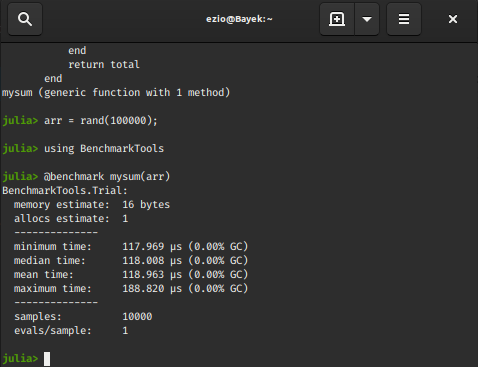Using SIMD macro:

 `function mysum1(arr::Vector)``    ``total ``=` `zero(eltype(arr))``    ``@simd` `for` `x ``in` `arr``        ``total ``+``=` `x``    ``end``    ``return` `total``end`` ` `# benchmark the mysum1 function``@benchmark` `mysum1(arr)`

Output: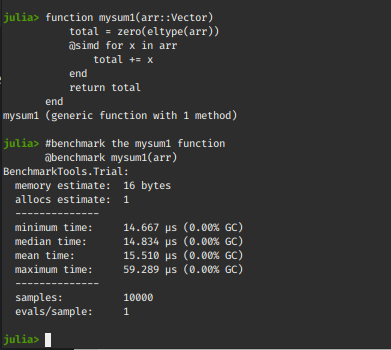We can clearly see the performance increase after using the SIMD macro.
So what’s actually happening ?
By taking advantage of the SIMD instruction set(inbuilt feature of most Intel® CPU’s), the add operation is performed in two steps:

During the first step, intermediate values are accumulated n at a time (n depends on the CPU hardware).
In a so called reduction step, the final n elements are summed together.

Now the question arises, how can we combine SIMD vectorization and the language’s vectorization capabilities to derive more performance both from the language’s compiler and the CPU? In Julia we answer it with the LoopVectorization.jl package.

### LoopVectorization

LoopVectorization.jl is Julia package that provides macros and functions that combine SIMD vectorization and loop reordering so as to improve performance.

#### @avx macro

It annotates a for loop, or a set of nested for loops whose bounds are constant across iterations, to optimize the computation.
Let’s consider the classical dot product problem. To know more about dot product and it’s vectorized implementation in python, check out this article.
In the below examples we will benchmark the same code with different types of vectorization.
Example:

 `# Without using any macro``function dotProd(x, y)``           ``prod ``=` `zero(eltype(x))``           ``for` `i ``in` `eachindex(x, y)``               ``prod ``+``=` `x[i] ``*` `y[i]``           ``end``           ``prod``end`` ` `# using the simd macro``function dotProd_simd(x, y)``           ``prod ``=` `zero(eltype(x))``          ``@simd` `for` `i ``in` `eachindex(x, y)``               ``prod ``+``=` `x[i] ``*` `y[i]``           ``end``           ``prod``end`` ` `# using the avx macro``using LoopVectorization``function dotProd_avx(x, y)``           ``prod ``=` `zero(eltype(x))``           ``@avx` `for` `i ``in` `eachindex(x, y)``               ``prod ``+``=` `x[i] ``*` `y[i]``           ``end``           ``prod``end`

Output :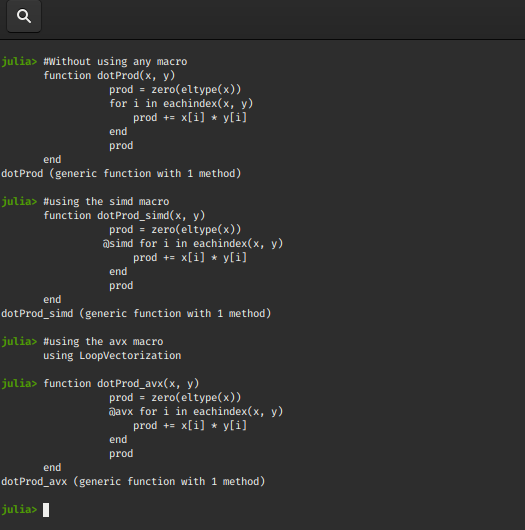Comparing the three functions:

 `using BenchmarkTools`` ` `# generating random data``x ``=` `rand(``10` `^ ``5``);``y ``=` `rand(``10` `^ ``5``);`` ` `# benchmark the function without any macro``@benchmark` `dotProd(x, y)   `` ` `# benchmark the function with simd macro``@benchmark` `dotProd_simd(x, y)`` ` `# benchmark the function with avx macro``@benchmark` `dotProd_avx(x, y)`

Output :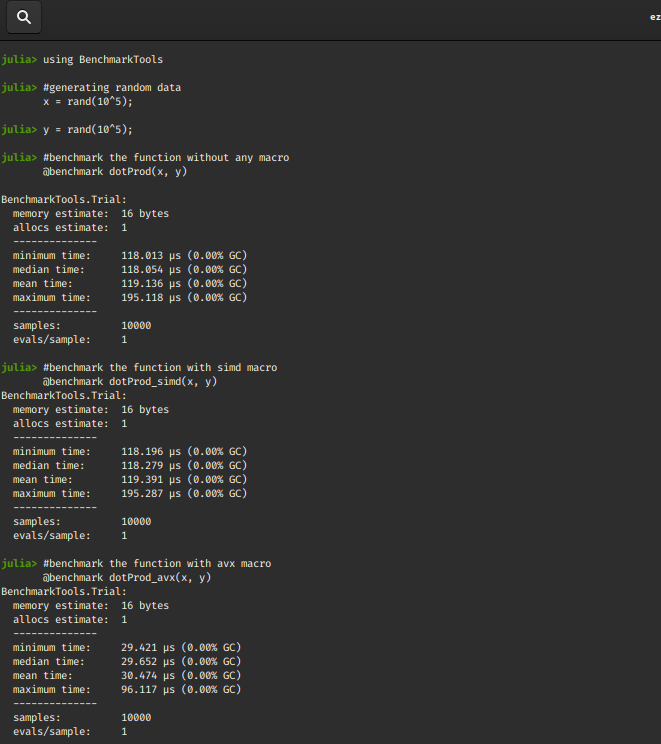We observe that the @avx macro turns out to have the best performance! The time gap will generally increase for larger sizes of x and y.

### Vectorized convenience functions

#### vmap

This is simply a SIMD-vectorized map function.
Syntax :

vmap!(f, destination_variable, x::AbstractArray)
vmap!(f, destination_varaible, x::AbstractArray, yb::AbstractArray, …)

It applies f to each element of x (or paired elements of x, y, …) and storing the result in destination-variable.
If the ! symbol is removed a new array is returned instead of the destination_variable.
vmap(f, x::AbstractArray)
vmap(f, x::AbstractArray, yb::AbstractArray, …)

Example:

 `# the function f``f(x, y) ``=` `1` `/` `(``1` `+` `exp(x``-``y))`` ` `# generate random data``x ``=` `rand(``10``^``5``);``y ``=` `rand(``10``^``5``);`` ` `# destination variable of the same dims as x``z ``=` `similar(x);`

Output :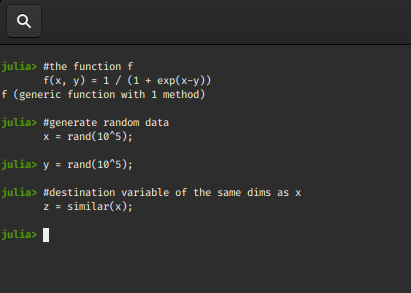`# benchmark the map function``@benchmark` `map``!(f, \$z, \$x, \$y)`` ` `# benchmark the vmap function``@benchmark` `vmap!(f, \$z, \$x, \$y)`

Output :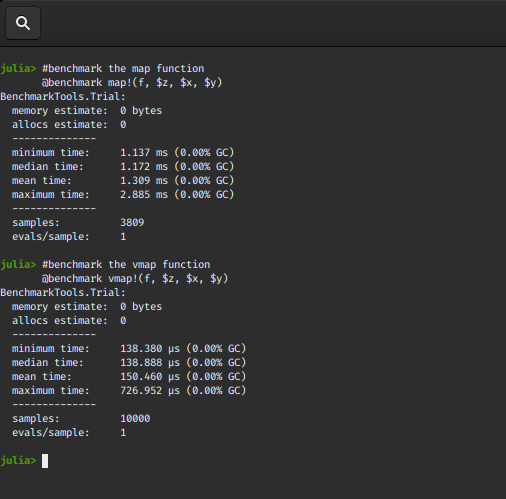We again observe that the vectorized map function performs quite faster.
Let’s look at some tweaked versions of the vmap function.

#### vmapnt

It is variant of vmap that can improve performance where the destination_variable is not needed during the vmap process.
Syntax :

```vmapnt(f, x::AbstractArray)
vmapnt(f, x::AbstractArray, y::AbstractArray, ...)

vmapnt!(f, destination_variable, x::AbstractArray, y::AbstractArray, ...)
```

This is a vectorized map implementation using nontemporal store operations.
It means if the arguments are very long(like in the previous example), the write operations
to the destination_variable will not go to the CPU’s cache. If we will not immediately be reading from these values, this can improve performance because the writes won’t pollute the cache.

#### vmapntt

It is a threaded variant of vmapnt.
Syntax :

```vmapntt(f, x::AbstractArray)
vmapntt(f, x::AbstractArray, y::AbstractArray, ...)
```

#### vmapntt!

Syntax :

```vmapntt!(f, destination_variable, x::AbstractArray, y::AbstractArray, ...)
```

#### vfilter

It is a SIMD-vectorized filter, returning an array containing the elements of x for which f returns true.
Syntax :

```vfilter(f, x::AbstractArray)
```

#### vfilter!

It is a SIMD-vectorized filter!, removing the element of x for which f is false.
Syntax :

```vfilter(f, x::AbstractArray)
```

Examples:

 `# benchmark the vmapnt function``@benchmark` `vmapnt!(f, \$z, \$x, \$y)`` ` `# change the number of threads``Threads.nthreads()``Threads.nthreads() ``=` `8``Threads.nthreads()`` ` `# benchmark the vmapntt function``@benchmark` `vmapntt!(f, \$z, \$x, \$y)`` ` `# benchmark the filter against vfilter construct``@benchmark` `filter``(a ``-``> a < ``0.67``, \$x)``@benchmark` `vfilter(a ``-``> a < ``0.67``, \$x)`

Output: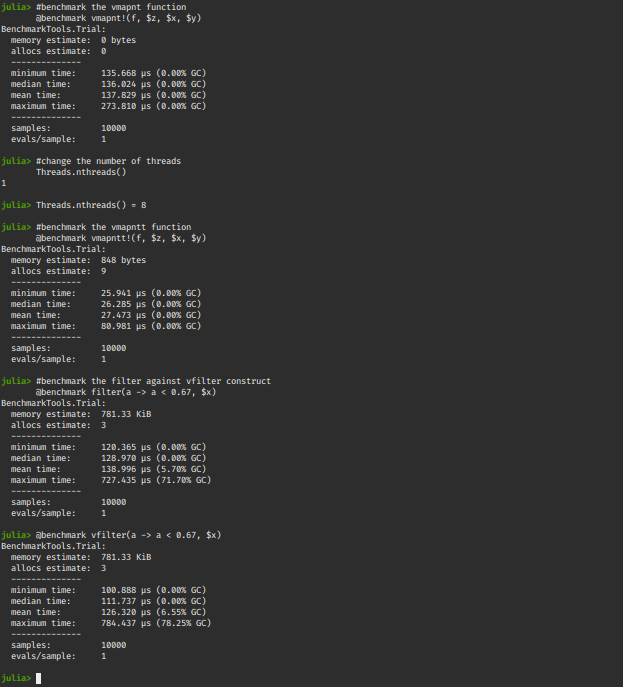My Personal Notes arrow_drop_up